# Python Zip() Function Tutorial With Code Examples

In Python, we can use the zip() function to aggregate or combine two iterables such as strings, lists, tuples, etc. The zip() function essentially “zips” together the iterables by returning a tuple containing each iterable’s corresponding elements.

The zip() function accepts one or more iterables, i.e., string, list, sets, and returns an iterator of tuples where each tuple contains elements from each iterable, which are passed into the zip() method.

Let’s now deep dive into what is zip() function and how you can use it to solve real-world problems.

## Syntax of Python Zip() function

Syntax: `zip(iterable1, iterable2, .......)`

Parameters: Iterables – Any iterable, i.e., string, list, tuple, etc.

It returns a zip object which works as an iterator.

Code Example:

```Books=['Book1','Book2','Book3']
Price=[500,200,300]
Pages=[999,499,500]

itr=zip(Books,Price,Pages)
print(list(itr))```

Output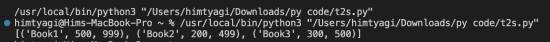From the output, we can notice that the resultant zip object has n number of tuples and the nth tuple contains the corresponding nth element from input iterables.

Let’s consider the two lists- x=[x1,x2,x3,…,xn] , y=[y1,y2,y3,…,yn]

zip_itr=zip(x,y)

Then, zip_itr=[(x1,y1),(x2,y2),…,(xn,yn)]

The zip object can be converted into a list of tuples dictionary using the below code.

Code Example:

```Books=['Book1','Book2']
Author=['Akhil','Himanshu']

itr1=zip(Books,Author)
# list of tuples
print(list(itr1))

itr2=zip(Books,Author)
# dictionary
print(dict(itr2))```

Output: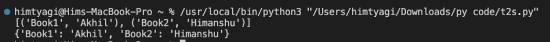## Iterating the zip object

We can traverse the elements in the zip object using the next() function until the StopIteration exception is caught.

Code Example:

```Books=['Book1','Book2']
Author=['Akhil','Himanshu']

zipObj=zip(Books,Author)

while(1):
try:
itr=next(zipObj)
print(itr,'-',itr)
except StopIteration:
break```

Output:## Passing arguments of unequal length

There are a few scenarios when we pass iterables of unequal length to the zip object. Below is such a case.

Code Example:

```Books=['Book1','Book2','Book3']
Price=[500,200]
Pages=

itr=zip(Books,Price,Pages)
print(list(itr))```

Output: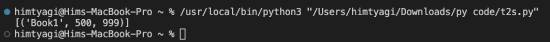## zip_longest() function

Here the zip iterator collects elements from each list till elements in the shortest length list are exhausted.

If we want to include unmatched elements from the other two lists in the zipped object, then use the zip_longest() method, defined in the itertools module.

Code Example:

```# import itertools module
import itertools

Books=['Book1','Book2','Book3']
Price=[500,200]
Pages=

itr=itertools.zip_longest(Books,Price,Pages)
print(list(itr))```

Output: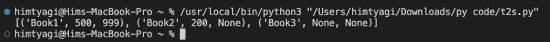In the above output, we can see that there are None values. We can replace these None values with the required ones using the fillvalue parameter in the zip_longest() method.

Code Example:

```# import itertools module
import itertools

Books=['Book1','Book2','Book3']
Price=[500,200]
Pages=

itr=itertools.zip_longest(Books,Price,Pages,fillvalue='-')
print(list(itr))```

Output: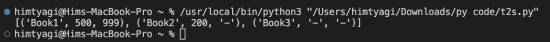## Applications or Usage of Zip() Function

### 1. Sorting the data in the zip object, which is formed with more than one iterable

Let’s consider two lists: Books=[‘Book1′,’Book2′,’Book3’], Price=[500,200,300].

We can sort the zip object formed using the above lists based on price by passing the Price list as the first iterable into the zip function and using the sorted() function.

Code Example:

```Books=['Book1','Book2','Book3']
Price=[500,200,300]

itr=zip(Price,Books)

print(sorted(list(itr)))```

Output: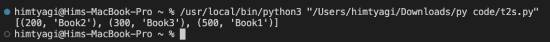### 2. Data Processing

We can perform some data calculations on the corresponding elements in iterables.

```Itemcost=[100,200,300]
Tax_percent=[10,20,30]

itr=zip(Itemcost,Tax_percent)

for itr1,itr2 in itr:
tax_amount=itr1*itr2/100
print('Itemcost-',itr1,'Tax_percent-',itr2,'Tax Amount-',tax_amount)```

Output: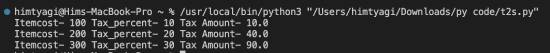### 3. Create a dictionary using two lists

You can also use Python’s zip() function to create a dictionary from two lists. Here’s an example of the same:

```keys = ['a', 'b', 'c']
values = [1, 2, 3]
d = dict(zip(keys, values))
print(d)```

Please note that if the length of both lists is unequal, then the zip() function will only combine the elements up to the length of the shortest length.

### 4. Iterate dictionaries

Another use case of the zip() function is to iterate over key and value pairs of dictionaries. Here’s a code example of this scenario:

```d = {'a': 1, 'b': 2, 'c': 3}
for key, value in d.items():
print(f'{key}: {value}')```

## Unzipping the Zip object

To unzip the zipped object, specify * before the zipped object in the zip() method. Below is a simple example program to unzip the zipped object.

Code Example:

```Books=['Book1','Book2']
Author=['Akhil','Himanshu']

zip_obj=zip(Books,Author)

# unzipping
itr1,itr2=zip(*zip_obj)

print(itr1)
print(itr2)```

Output: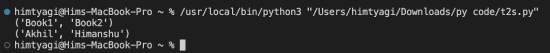## Python Zip() Function Examples

### 1. Zip two lists

We will use the Python zip() function to combine two lists in this example.

```a = [1, 2, 3]
b = ['a', 'b', 'c']
zipped = zip(a, b)
print(zipped)```

You can use the list() method in the above example to create zipped tuples.

### 2. Zip two dictionaries

Here we will create a list of tuples from two dictionaries, where each tuple consists of a key-value pair. Here’s an example:

```dict1 = {'a': 1, 'b': 2, 'c': 3}
dict2 = {'d': 4, 'e': 5, 'f': 6}

zipped_dicts = zip(dict1, dict2)
print(list(zipped_dicts))```

### 3. Zip for loop

Here we will use for loop to iterate over the tuples returned by the zip() function. Let’s understand it with a code example:

```dict1 = {'a': 1, 'b': 2, 'c': 3}
dict2 = {'d': 4, 'e': 5, 'f': 6}

for key1, key2 in zip(dict1, dict2):
print(key1, key2)```

### 4. Zip() 3 lists

You can use the zip function to iterate over multiple lists simultaneously. To iterate over three lists, you can use the zip function in combination with the for loop like this:

```list1 = [1, 2, 3]
list2 = [4, 5, 6]
list3 = [7, 8, 9]

zipped_lists = list(zip(list1, list2, list3))
print(zipped_lists)```